Get Started »

# Discrete Mathematics

 In:=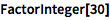⨯ `FactorInteger`
 Out=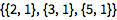Find the GCD (or LCM) of any two integers:

 In:=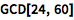⨯ `GCD[24, 60]`
 Out=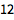Display the 4`th prime number:`

``` ```
``` In:=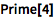⨯ Prime Out=Test the primality of a number: In:=⨯ PrimeQ[%] Out=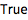This works with coprime numbers as well: In:=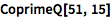⨯ CoprimeQ[51, 15] Out=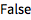```
``` Use the Mod function for the remainder: In:=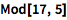⨯ Mod[17, 5] Out=Get all possible permutations of a list: In:=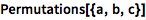⨯ Permutations[{a, b, c}] Out=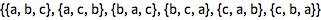Apply Permute to a list using disjoint Cycles: (Cycles takes a list of lists as an argument.) In:=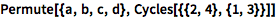⨯ Permute[{a, b, c, d}, Cycles[{{2, 4}, {1, 3}}]] Out=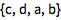Find the permutation order: In:=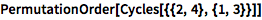⨯ PermutationOrder[Cycles[{{2, 4}, {1, 3}}]] Out=Generate a Graph from a list of edges: (Use ESCueESC for an UndirectedEdge or ESCdeESC for a DirectedEdge.) In:=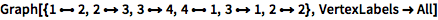⨯ Graph[{1 <-> 2, 2 \[DirectedEdge] 3, 3 \[DirectedEdge] 4, 4 <-> 1, 3 \[DirectedEdge] 1, 2 \[DirectedEdge] 2}, VertexLabels -> All] Out=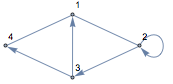Find the shortest path between two vertices: In:=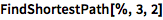⨯ FindShortestPath[%, 3, 2] Out=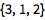Explore well-known graphs using natural-language input: In:=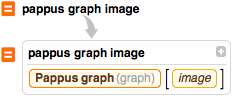X pappus graph image Out=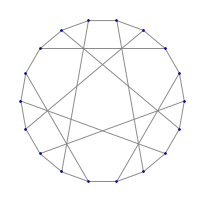The Wolfram Language also includes functions for combinatorics, probability, integer sequences and much more. QUICK REFERENCE: Number Theoretic Functions » QUICK REFERENCE: Discrete Mathematics »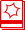Hands–on Start toWolfram Mathematica »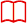Full Documentation »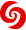Demonstrations Project » ```
``` ```
``` › ```
``` ```
``` ```
``` ```
``` Enable JavaScript to interact with content and submit forms on Wolfram websites. Learn how » ```
``` Deutsch English Español Français 日本語 한국어 Português Русский 中文 ```